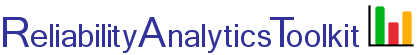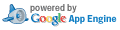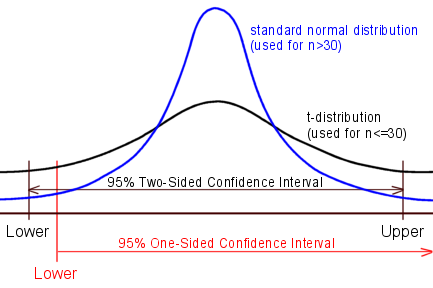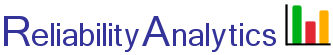# Confidence Limits - Normal Distribution

This tool calculates confidence intervals around point estimates of mean life for items whose lifetimes are assumed to be normally distributed. For sample sizes greater than 30 the standard Normal distribution is used. For smaller sample sizes (n<30) the t-distribution is used to calculate confidence (ref. 1). As an option, check item 4 and enter failure times into box 4 to have the tool calculate inputs 1-3. The tool is pre-filled with example test data (ref. 2 data multiplied by 100).## Calculation Inputs:

1. Mean life (M, hours):
2. Standard deviation (s, hours):
3. Sample size (n):
4. Optional: Use failure times to calculate M, s, n (one value per line, example failure times)
5. Confidence level:
6. Decimal places:

## Featured Reference:Practical Reliability Engineering

Toolkit Home